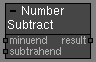# Number Subtract node

Left Toolbar >Math Nodes >Number Subtract

The Number Subtract node allows you to execute a subtraction operation.

## Procedure

### To execute a subtraction operation in the node graph:

1. On the left toolbar choose Math Nodes> Number Subtract2. Drag the Number Subtract icon into the Node Graph.3. Connect the Output: Result.

## Inputs

Minuend First number from which the second number is to be subtracted. A NUMBER type of value.

Subtrahend Second number, is the number to be subtracted from minuend. A NUMBER type of value.

## Outputs

Result Difference = Minuend - Subtrahend.

## Attributes PanelMinuend First value in the subtraction operation. This attribute is exposed as input by default, but it can be defined in the attributes panel.

Subtrahend Second value in the subtraction operation. This attribute is exposed as input by default, but it can be defined in the attributes panel.

Math Nodes

Main Page